## Unsigned and Signed Binary Numbers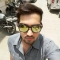Updated on 30-Jul-2019 22:30:24
Variables such as integers can be represent in two ways, i.e., signed and unsigned. Signed numbers use sign flag or can be distinguish between negative values and positive values. Whereas unsigned numbers stored only positive numbers but not negative numbers.Number representation techniques like: Binary, Octal, Decimal and Hexadecimal number representation techniques can represent numbers in both signed and unsigned ways. Binary Number System is one the type of Number Representation techniques. It is most popular and used in digital systems. Binary system is used for representing binary quantities which can be represented by any device that has only two operating ... Read More

## Arithmetic of Number Systems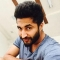Updated on 30-Jul-2019 22:30:24
A number system is a set of symbols used to represent values derived from a common base or radix. In a number, the value of each digit can be determined using digit, position of the digit in the number, and the base of the number system. The base is defined as the total number of digits are available in the number system. This is known as positional number system.Number SystemBaseDigit UsedBinary20, 1Octal80, 1, 2, 3, 4, 5, 6, 7Decimal100, 1, 2, 3, 4, 5, 6, 7, 8, 9Hexadecimal160, 1, 2, 3, 4, 5, 6, 7, 8, 9, A, B, C, D, ... Read More

## Bubble Sort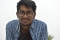Updated on 15-Jun-2020 14:46:51
Bubble Sort is a comparison based sorting algorithm. In this algorithm adjacent elements are compared and swapped to make the correct sequence. This algorithm is simpler than other algorithms, but it has some drawbacks also. This algorithm is not suitable for a large number of data set. It takes much time to solve the sorting tasks.The complexity of the Bubble Sort TechniqueTime Complexity: O(n) for best case, O(n^2) for average and worst caseSpace Complexity: O(1)Input and OutputInput: A list of unsorted data: 56 98 78 12 30 51 Output: Array after Sorting: 12 30 51 56 78 98AlgorithmbubbleSort( array, size)Input ... Read More

## Jump Search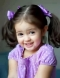Updated on 15-Jun-2020 14:00:54
Jump search technique also works for ordered lists. It creates a block and tries to find the element in that block. If the item is not in the block, it shifts the entire block. The block size is based on the size of the list. If the size of the list is n then block size will be √n. After finding a correct block it finds the item using a linear search technique. The jump search lies between linear search and binary search according to its performance.The complexity of Jump Search TechniqueTime Complexity: O(√n)Space Complexity: O(1)Input and OutputInput: A sorted ... Read More

## Binary Search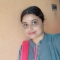Updated on 15-Jun-2020 14:14:41
When the list is sorted we can use the binary search technique to find items on the list. In this procedure, the entire list is divided into two sub-lists. If the item is found in the middle position, it returns the location, otherwise jumps to either left or right sub-list and do the same process again until finding the item or exceed the range.The complexity of Binary Search TechniqueTime Complexity: O(1) for the best case. O(log2 n) for average or worst case.Space Complexity: O(1) Input and OutputInput:  A sorted list of data: 12 25 48 52 67 79 88 93 The ... Read More